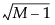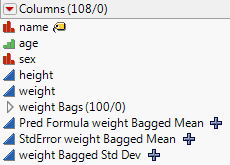Profilers > Profiler > Bagging
Publication date: 05/24/2021

# Bagging

Bootstrap aggregating (bagging) is a technique to improve predictive performance while also gaining insight into the reliability of predictions. Bagging is especially useful in unstable methods, including neural networks, classification trees, and regression trees.

Bagging creates M training data sets by sampling with replacement from the original data. All training data sets are of the same size as the original. For each training data set, a model is fit using the analysis platform, and predictions are made. Therefore, there are a total of M predictions for each observation in the original data set. The final prediction is the average of the M predictions.

Bagging is available in many analysis platforms. To use bagging, select Save Bagged Predictions from the Prediction Profiler red triangle menu. A window appears with the following options for Bagging:

Number of Bootstrap Samples

Sets the number of times that you want to resample the data and build a model. A larger number results in more precise predictions. By default, the number of bootstrap samples is 100.

Random Seed

Sets a random seed that you can re-enter in subsequent runs of the bagging analysis to duplicate your current results. By default, no seed is set.

Fractional Weights

Performs a Bayesian bagging analysis. In each bootstrap iteration, each observation is assigned a nonzero weight. The model that makes the predictions uses the weighted observations. By default, the Fractional Weights option is not selected, and a simple bagging analysis is conducted.

Tip: Use the Fractional Weights option if the number of observations that are used in your analysis is small or if you are concerned about separation in a logistic regression setting.

Suppose that Fractional Weights is selected. For each bootstrap iteration, each observation that is used in the report is assigned a nonzero weight. These weights sum to n, the number of observations used in the model. For more information about how the weights are calculated and used, see Calculation of Fractional Weights in Basic Analysis.

Save Prediction Formulas

For each bagged prediction, this option saves the formula used to make that prediction in the column properties. This option is available in only a subset of the analysis platforms that offer bagging.

Note: If Save Prediction Formulas is not available, a note appears, stating that only the predicted values will be saved.

Bagging automatically creates new columns in the original data table. All M sets of bagged predictions are saved as hidden columns. The final prediction is saved in a column named “Pred Formula <colname> Bagged Mean”. The standard deviation of the final prediction is saved in a column named “<colname> Bagged Std Dev”. The standard error of the bagged mean is saved in a column named “StdError <colname> Bagged Mean.” The standard error is the standard deviation divided by. Here, <colname> identifies the column in the report that was bagged.

The standard error gives insight about the precision of the prediction. A very small standard error indicates a precise prediction for that observation. For more information about bagging, see Hastie et al. (2009).

Figure 3.17 Bagging Columns